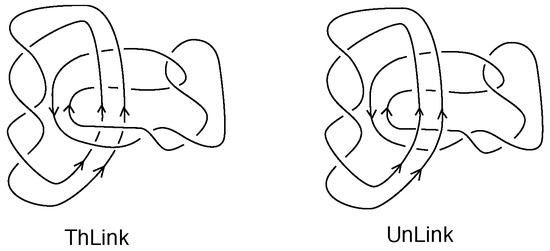Next Article in Journal
Asymmetric Equivalences in Fuzzy Logic
Next Article in Special Issue
Open Gromov-Witten Invariants from the Augmentation Polynomial
Previous Article in Journal
Reversible Dual-Image-Based Hiding Scheme Using Block Folding Technique
Previous Article in Special Issue
On the Fibration Defined by the Field Lines of a Knotted Class of Electromagnetic Fields at a Particular Time
Open AccessFeature PaperArticle

# Skein Invariants of Links and Their State Sum Models

1
Department of Mathematics, Statistics and Computer Science, University of Illinois at Chicago, Chicago, IL 60607-7045, USA
2
School of Applied Mathematical and Physical Sciences, National Technical University of Athens, 15780 Athens, Greece
*
Author to whom correspondence should be addressed.
These authors contributed equally to this work.
Symmetry 2017, 9(10), 226; https://doi.org/10.3390/sym9100226
Received: 19 September 2017 / Revised: 2 October 2017 / Accepted: 9 October 2017 / Published: 13 October 2017
(This article belongs to the Special Issue Knot Theory and Its Applications)
We present the new skein invariants of classical links, $H [ H ]$ , $K [ K ]$ and $D [ D ]$ , based on the invariants of links, H, K and D, denoting the regular isotopy version of the Homflypt polynomial, the Kauffman polynomial and the Dubrovnik polynomial. The invariants are obtained by abstracting the skein relation of the corresponding invariant and making a new skein algorithm comprising two computational levels: first producing unlinked knotted components, then evaluating the resulting knots. The invariants in this paper, were revealed through the skein theoretic definition of the invariants $Θ d$ related to the Yokonuma–Hecke algebras and their 3-variable generalization $Θ$ , which generalizes the Homflypt polynomial. $H [ H ]$ is the regular isotopy counterpart of $Θ$ . The invariants $K [ K ]$ and $D [ D ]$ are new generalizations of the Kauffman and the Dubrovnik polynomials. We sketch skein theoretic proofs of the well-definedness and topological properties of these invariants. The invariants of this paper are reformulated into summations of the generating invariants (H, K, D) on sublinks of the given link L, obtained by partitioning L into collections of sublinks. The first such reformulation was achieved by W.B.R. Lickorish for the invariant $Θ$ and we generalize it to the Kauffman and Dubrovnik polynomial cases. State sum models are formulated for all the invariants. These state summation models are based on our skein template algorithm which formalizes the skein theoretic process as an analogue of a statistical mechanics partition function. Relationships with statistical mechanics models are articulated. Finally, we discuss physical situations where a multi-leveled course of action is taken naturally. View Full-Text
Show FiguresFigure 1

MDPI and ACS Style

Kauffman, L.H.; Lambropoulou, S. Skein Invariants of Links and Their State Sum Models. Symmetry 2017, 9, 226.

AMA Style

Kauffman LH, Lambropoulou S. Skein Invariants of Links and Their State Sum Models. Symmetry. 2017; 9(10):226.

Chicago/Turabian Style

Kauffman, Louis H.; Lambropoulou, Sofia. 2017. "Skein Invariants of Links and Their State Sum Models" Symmetry 9, no. 10: 226.

Find Other Styles
Note that from the first issue of 2016, MDPI journals use article numbers instead of page numbers. See further details here.

### Article Access Map by Country/Region

1
Search more from Scilit